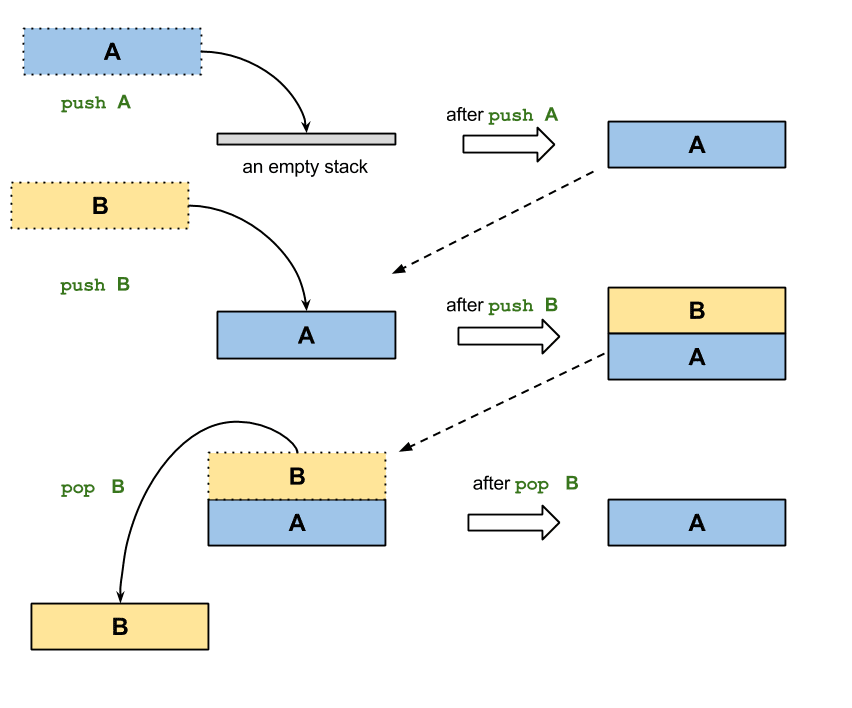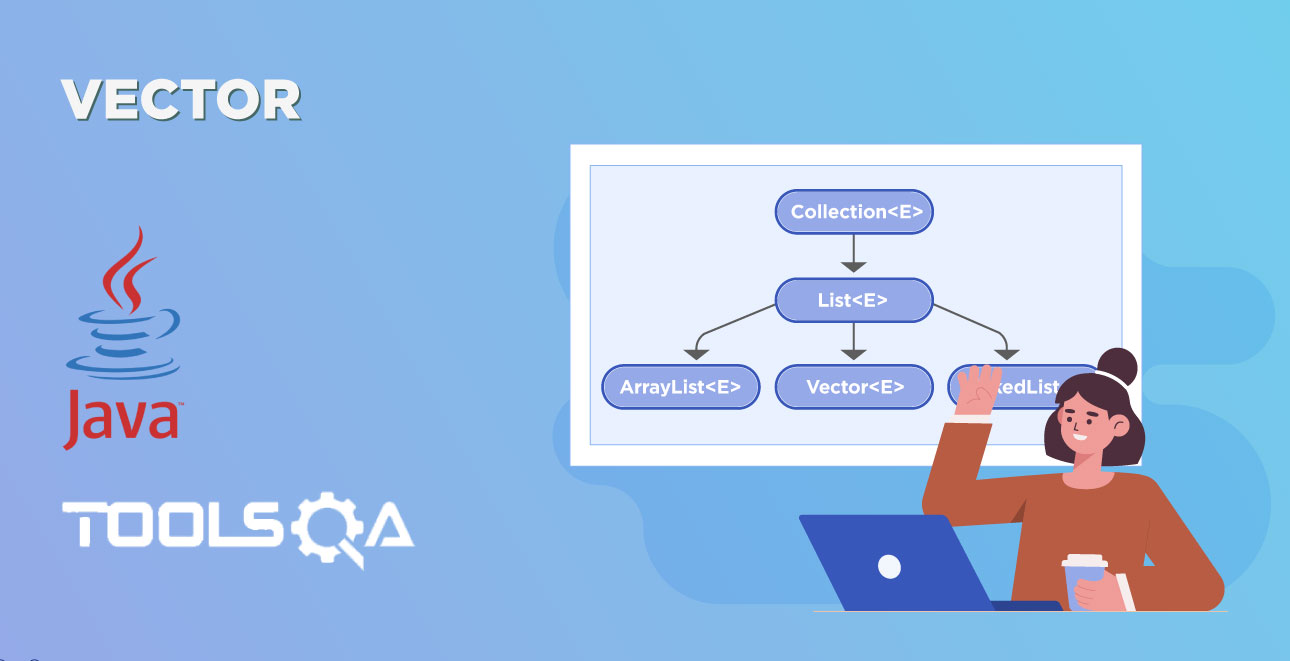## Stack

In programming, a stack is a collection of objects that serve two principal operations:

• push: add an element to the collection
• pop: get and remove the most recently pushed elementThe Stack class represents a last-in-first-out (LIFO) stack of objects. While a Vector starts index at 0, a Stack starts index at 1. It extends class Vector with five operations that allow a vector to be treated as a stack. The additional operations are:

``````// Tests if this stack is empty.
boolean empty();

// Read the object at the top of this stac
E   peek()

// Retrieve and remove the object at the top of this stack
E   pop()

// Pushes an item onto the top of this stack.
E   push(E item)

// Find the position of an object in this stack
int search(Object o)
``````

### Example

We demonstrate how peek(), pop, push behave in the following example. Be sure to check if the stack is empty() before calling peek() or pop() to avoid the EmptyStackException exception.

``````import java.util.Stack;

public class StackTest {
public static void printStack(Stack<String> words) {
System.out.print("[");
while(!words.empty()) {
System.out.print(words.pop() + " ");
}
System.out.println("]");
}

public static void find(Stack<String> words, String w) {
int idx = words.search("thrive");
if (idx < 0) {
} else {
System.out.println("Index of [" + w + "] is: " + idx);
}
}

public static void main(String []args) {
Stack<String> words = new Stack<String>();
// append
words.push("thrive");
words.push("at");
words.push("work");
System.out.println("Peek is: [" + words.peek() + "]");

System.out.println("> Before pop ");
find(words, "thrive");
printStack(words);
System.out.println("> After pop ");
find(words, "thrive");
}

}
``````

The above code produces:

Peek is: [work]

>Before pop Index of [thrive] is: 3 [work at thrive ]